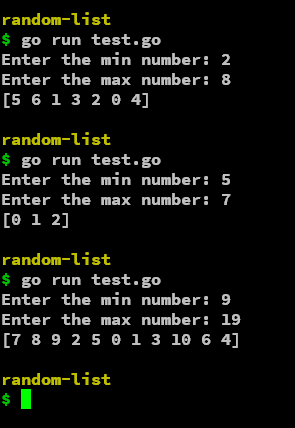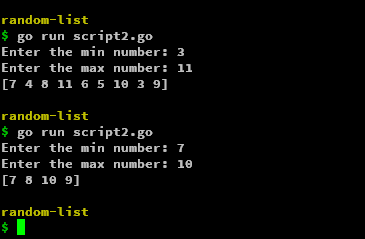# How to Generate a Array of Unique Random Numbers in Golang?

• Last Updated : 03 Jun, 2022

Sometimes we need some random numbers but not repeated, generally random numbers are pseudo-random and there is definitely a chance of repetition in them. Let’s say we have a range of numbers and we want to have n number of elements in a shuffled way between that range.

### Using rand.Perm

Simply we will begin by creating a file by any appropriate name and with a .go extension. We will be using the math random module in Golang, so we need to import that module.

## Go

 `// Go program generating an array of unique random numbers ` `package` `main`   `import` `(` `    ``"fmt"` `    ``"math/rand"` `)`   `func` `main() {` `    ``permutation := rand.Perm(``5``)`   `    ``fmt.Println(permutation)` `}`

Output:

`[0 4 2 3 1]`

As we can see it gives a permutation to arrange five objects that are random at once but it doesn’t change the order after every run or iteration. So we are not completely generating a random list, we need to have a different order of permutation each time. So, to do that, we use the random module with the time module. We need to generate a different seed in order for the permutation function to shuffle the order. By default, the seed remains the same, but if we add the current time as a seed it produces a different seed and in turn, generates the order accordingly.

To add that level of randomness, we need to use the time module, inside of that we need to get the current time, which can be obtained by using time.Now(), this gives the current time in the date-time format. This format cannot be used as a seed, so we convert it to seconds using the Unix function. The Unix function gives the seconds since 1st Jan 1970. You can even use UnixNano, UnixMicro, or UnixMill. These are just returning the Unix seconds in nano, micro, and milliseconds.

rand.Seed(time.Now().Unix())

So, if we add the following command to the script, the permutation function will generate the order randomly.

## Go

 `// Go program generating an array of unique random numbers ` `package` `main`   `import` `(` `    ``"fmt"` `    ``"math/rand"` `    ``"time"` `)`   `func` `main() {` `    ``rand.Seed(time.Now().Unix())` `    ``permutation := rand.Perm(``5``)`   `    ``fmt.Println(permutation)` `}`

Output:

`[4 0 1 2 3]`

### Defining a Range For Numbers in the List

To define the ranges, we can input those from the user or just set them at a fixed value. The permutation function however won’t allow entering the minimum and maximum range, It simply returns n numbers in order of range from 0 to n-1 provided n as a parameter to the function.

## Go

 `// Go program to define a range for numbers in the list` `package` `main`   `import` `(` `    ``"fmt"` `    ``"math/rand"` `    ``"time"` `)`   `func` `main() {` `    ``rand.Seed(time.Now().Unix())` `    ``min := ``3` `    ``max := ``7` `    ``permutation := rand.Perm(max - min + ``1``)`   `    ``fmt.Println(permutation)` `}`

Output:

`[3 4 0 1 2]`

We will use a min and a max variable to set the minimum and the maximum limit to generate the numbers. So to generate the numbers between the provided minimum and maximum range, we can pass max-min+1 to the function as it would generate the required numbers. We are adding one because the range is inclusive. For example, here the min value is 3 and the max value is 7, we clearly have 5 values to pick from i.e. 3,4,5,6,7 so the formula fits perfectly max-min+1. So, we have 5 numbers and give the number 5 as the parameter to the Permutation function and it will render numbers 0 to 5-1 in random order i.e. [0 1 2 3 4] in randomized order.

To test this, we can add user input for min-max and see the permutation function display the numbers.

## Go

 `// Go program to take user input for min-max and see the ` `// permutation function display the numbers` `package` `main`   `import` `(` `    ``"fmt"` `    ``"math/rand"` `    ``"time"` `)`   `func` `main() {` `    ``rand.Seed(time.Now().Unix())` `    ``var` `min int` `    ``var` `max int` `    ``fmt.Print(``"Enter the min number: "``)` `    ``fmt.Scan(&min)` `    ``fmt.Print(``"Enter the max number: "``)` `    ``fmt.Scan(&max)` `    ``permutation := rand.Perm(max - min + ``1``)`   `    ``fmt.Println(permutation)` `}`

Output:So, we can see the number of elements between the range is getting arranged with the help of the permutation function. No, we will look into how to have these values actually between the minimum and maximum range.

### Creating an Array with Permutation from min to max

To get the element’s value between the minimum and maximum range, we simply need to add the minimum limit to all the elements. It will eventually get accommodated in the given range.

for i := range permutation {

random_list[i] += min

}

So, this basically shifts every element by the minimum value so automatically the numbers get in the range between (minimum, maximum). After we add that in the script, it finally becomes as follows:

## Go

 `// Go program for creating an array with permutation from min to max` `package` `main`   `import` `(` `    ``"fmt"` `    ``"math/rand"` `    ``"time"` `)`   `func` `main() {` `    ``rand.Seed(time.Now().Unix())` `    ``var` `min int` `    ``var` `max int` `    ``fmt.Print(``"Enter the min number: "``)` `    ``fmt.Scan(&min)` `    ``fmt.Print(``"Enter the max number: "``)` `    ``fmt.Scan(&max)` `    ``random_list := rand.Perm(max - min + ``1``)`   `    ``for` `i := ``range` `random_list {` `        ``random_list[i] += min` `    ``}`   `    ``fmt.Println(random_list)` `    ``/*` `    ``for _, num := range random_list {` `        ``fmt.Println(num)` `    ``}` `    ``*/` `}`

The code in the comments is basically iterating over the generated array i,e, the unique random list, and prints the elements one by one.My Personal Notes arrow_drop_up
Recommended Articles
Page :